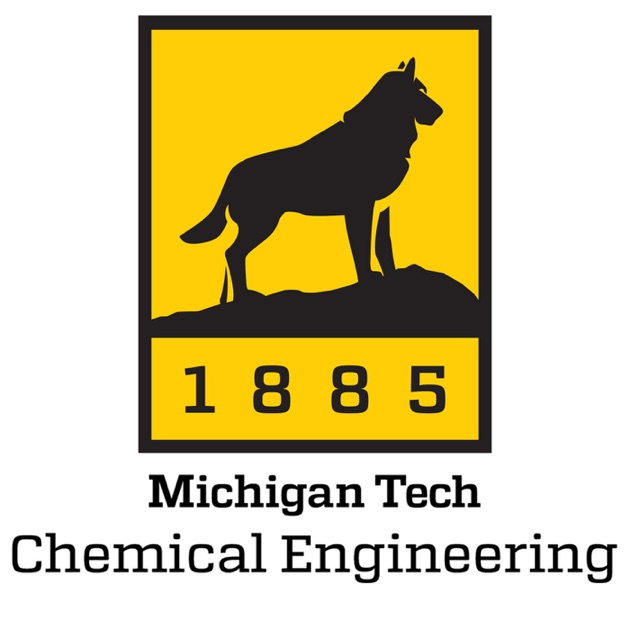Course Materialsche 31. Introduction To Chemical Engineering

Introduction to chemical engineering

Lecturer(s) :

Hatzimanikatis Vassily

Course Materialsche 31. Introduction To Chemical Engineering Processes

English

Summary

Introduction to Chemical Engineering is an introductory course that provides a basic overview of the chemical engineering field. It addresses the formulation and solution of material and energy balances by using the physical/chemical properties of materials.

Content

Basis concepts

• Definition of chemical engineering
• Definition of steady-state and transient system
• Introduction to mass balances
• Introduction to energy balances
• introduction to combined mass and energy balance

Keywords

Flowchart of chemical process

Mass balance

Energy balance

Degree of freedom analysisUnit operations

Learning Prerequisites

Required courses

General chemistry

Physics

Algebra

Important concepts to start the course

an understanding of chemical and physical properties of materials

an ability to apply knowledge of mathematics to solve equations

Learning Outcomes

By the end of the course, the student must be able to:• Draw the flowchart of chemical processes for single and multiple unit operations and label all the streams
• Identify the process variables and develop relationships between process variables
• Analyze all the units by doing a degree of freedom (DOF) analysis
• Specify the reactive and non-reactive systems
• Formulate the mass and energy balances equations required to solve the system and calculate all the unknowns
• Use tables and charts to pick up physical property data needed to solve material and energy balances
• Report all the assumptions and engineering calculations and problem solutions in a stepwise manner

Transversal skills

• Set objectives and design an action plan to reach those objectives.
• Use a work methodology appropriate to the task.
• Access and evaluate appropriate sources of information.
• Give feedback (critique) in an appropriate fashion.

Teaching methods

The course is presented using powerpoint slides. In the 2 first hours of the course the concepts are introduced and several examples are shown and the students are asked to work together for few minutes and then sugest the solution methods. Finally the solutions of the examples are shown. In the third hour (excercise session), several problems are given to students. They work on problems and ask questions for 30 minutes and then the solutions are given by assistants on the blackboard for the rest 30 minutes.

Course Materialsche 31. Introduction To Chemical Engineering Flowchart

Taking notes in the course hours

Working in groups to solve the examples given in the course hours

Solve the problems in the exercise hour

Assessment methods

This course provides a continious evaluation of the students. There are 3 written exams including the final exam. The first exam is a bonus exam and the second is the midterm exam.

Resources

Bibliography

Elementary Principles of Chemical Processes, by Richard M. Felder & Ronald W. Rousseau. Wiley 2004.Notes/Handbook

Students have access to the material of the course (slides) few days before each course.